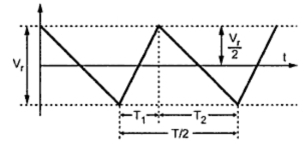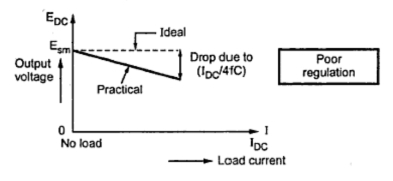### Capacitor Input Filter : Part3

1.3 Approximate Analysis of Capacitor Input Filter
Consider an output waveform for a full wave rectifier circuit using a capacitor input filter, as shown in the Fig. 1.Fig. 1 Derivation of ripple factor

Let      T = Time period of the a.c. input voltage
T/2 = Half of the time period
T1 = Time for which diode is conducting
T2 = Time for which diode is nonconducting.
During time T1, capacitor gets charged and this process is quick. During time T2, capacitor gets discharged through RL. As time constant RLC is very large, discharging process is very slow and hence T2 >> T1.
Let Vr be the peak to peak value of ripple voltage, which is assumed to be triangular as shown in the Fig. 2.Fig. 2  Triangle approximation of ripple voltage

It is known mathematically that the r.m.s. value of such a triangular waveform is,
During the time interval T2, the capacitor C is discharging through the load resistance RL. The charge lost is,
As integration gives average or d.c. value

But              IDC = EDC/R
For half wave rectifier with capacitor input filter the ripple factor is,
The product CRL is the time constant of the filter circuit.
From the expression of the ripple factor, it is clear that increasing the value of capacitor C, the ripple factor gets decreased. Thus the output can be made smoother, reducing the ripple content by selecting large value of capacitor. However very large value of capacitor can not be used because larger the value of capacitor, larger the initial charging surge current. This may exceed the current rating of the diodes in the rectifier. Otherwise the diodes used must be of higher current rating which increases the cost.
1.4 Why Capacitor Filter is not Suitable for Variable Loads ?
The another factor controlling the ripple factor is load resistance RL. As the load current drawn increases, for the same d.c. output voltage, the load resistance decreases. This increases the ripple contents in the output. Hence the filter is not suitable for the variable loads. The described features of the filter is high voltage and less ripple at the output for small load currents.
1.5 How to Decrease Ripple Factor ?
It can be seen from the expression of ripple factor that to decrease its value,
1. Increase the value of filter capacitor.
2. Increase the value of load resistance.
But higher C means larger initial surge current for which higher rating diodes must be used.
As RL decreases, the load current increases but ripple increases. Hence filter is not suitable for variable loads or higher loads. The capacitor filter is suitable for lighter loads i.e. small load currents.
1.6 D.C. Output Voltage with Capacitor Filter
The d.c. output voltage from a capacitor filter fed from a full wave rectifier is given by,
While the d.c. output voltage from a capacitor filter fed from a half wave rectifier is given by,
From the above expressions, it can be seen that as the current drawn by the load increases, the d.c. output voltage decreases. Hence this filter circuit is having poor regulation. The load regulation graph i.e. regulation characteristics for the capacitor input filter is shown in the Fig. 3.Fig . 3  Load regulation with capacitor input filter

1.7 Expression for Ripple Voltage
The ripple voltage present in the output can be obtained from the ripple factor.
Thus Er(r.m.s.)  = R.M.S. ripple voltage = EDC x Ripple factor
For ful wave rectifier,
Thus the ripple voltage with capacitor input filter can be obtained by multiplying ripple factor with d.c. output voltage or by using equation (14) if the d.c. load current current is known.
1.8 Surge Current in a Capacitor Input Filter
The capacitor has a feature that initially it acts as a short circuit momentarily. It does not offer any resistance.
The forward resistance of the diode is very small.
Hence at start, large current flows through the forward biased diode.
This is called surge current. Such an initial inrush of surge current can destroy the diodes. The diode current rating must be so as to withstanding high surge current.
The surge current is shown in the Fig. 4(a).
The surge current can be limited by connecting resistance Rs in series with the capacitor as shown in the Fig.4(b).Fig. 4

The advantages of capacitor input filter are,
1. Less number of components.
2. Low ripple factor hence low ripple voltage.
3. Suitable for high voltage at small load currents.
The advantages of capacitor input filter are,
1. Ripple factor depends on load resistance.
2. Not suitable for variable loads as ripple content increases as RL decreases.
3. Regulation is poor.
4. Diodes are subjected to high surge currents hence must be selected accordingly.

Examples on capacitor input filter